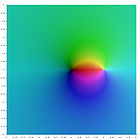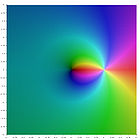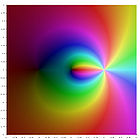﻿ Eulerian polynomials

# Eulerian polynomials

Peter Luschny,  2010−08−18

## Definition

The Eulerian polynomials are defined by the exponential generating function

The Eulerian polynomials can be computed by recurrence:

$A 0 ( t ) = 1 ,$ $A n ( t ) = t ( 1 − t ) A n − 1 ′ ( t ) + A n − 1 ( t ) ( 1 + ( n − 1 ) t ) ( n ≥ 1 ) .$

An equivalent way to write this definition is to set the Eulerian polynomials inductively by

$A 0 ( t ) = 1 ,$ $A n ( t ) = ∑ k = 0 n − 1 n k A k ( t ) ( t − 1 ) n − 1 − k ( n ≥ 1 ) .$

The definition given is used by major authors like D. E. Knuth, D. Foata and F. Hirzebruch. In the older literature (for example in L. Comtet, Advanced Combinatorics) a slightly different definition is used, namely

$C 0 ( t ) = 1 ,$ $C n ( t ) = t ( 1 − t ) C n − 1 ′ ( t ) + C n − 1 ( t ) ( n t ) ( n ≥ 1 ) .$

## Eulerian numbers

The coefficients of the Eulerian polynomials are the Eulerian numbers ${A}_{n,k}$ ,

This definition of the Eulerian numbers agrees with the combinatorial definition in the DLMF. The triangle of Eulerian numbers is also called Euler's triangle .

 An,k 0 1 2 3 4 0 1 0 0 0 0 1 1 0 0 0 0 2 1 1 0 0 0 3 1 4 1 0 0 4 1 11 11 1 0
 Cn,k 0 1 2 3 4 0 1 0 0 0 0 1 0 1 0 0 0 2 0 1 1 0 0 3 0 1 4 1 0 4 0 1 11 11 1

Euler's definition ${A}_{n,k}$ is A173018. The main entry for the Eulerian numbers in the database is A008292. It enumerates ${C}_{n,k}$ with offset (1,1).

## The combinatorial interpretation

Let ${S}_{n}$ denote the set of all bijections (one-to-one and onto functions) from $\left\{1,2,\dots ,n\right\}$ to itself, call an element of ${S}_{n}$ a permutation p and identify it with the ordered list ${p}_{1}{p}_{2}\dots {p}_{n}$ .

Using the Iverson bracket [.] the number of ascents of p is defined as

$asc ( p ) = ∑ i = 1 n p i < p i + 1 ,$

where ${p}_{n+1}←0$ . The combinatorial interpretation of the Eulerian polynomials is then given by

$A n ( x ) = ∑ p ∈ S n x asc ( p ) .$

The table below illustrates this representation for the case n = 4.

 p asc p asc p asc p asc 4321 0 4231 1 2413 2 1423 2 3214 1 2431 1 2134 2 1342 2 3241 1 4312 1 2314 2 4123 2 3421 1 3142 1 2341 2 1324 2 4213 1 4132 1 3124 2 1243 2 2143 1 1432 1 3412 2 1234 3

The number of permutations of  {1, 2, …, n} with n ascents (the central Eulerian numbers) are listed in A180056.

## HistoryEulerian polynomials in Institutiones calculi differentialis, 1755

Leonhard Euler introduced the polynomials in 1749  in the form

Euler introduced the Eulerian polynomials in an attempt to evaluate the Dirichlet eta function

$η ( s ) = ∑ n = 1 ∞ ( − 1 ) n − 1 n s$

at $s=-1,-2,-3,\dots$ . This led him to conjecture the functional equation of the eta function (which immediately implies the functional equation of the zeta function). Most simply put, the relation Euler was after was

Though Euler's reasoning was not rigorous by modern standards it was a milestone on the way to Riemann's proof of the functional equation of the zeta function.

The facsimile shows Eulerian polynomials as given by Euler in his work Institutiones calculi differentialis, 1755. It is interesting to note that the original definition of Euler coincides with the definition in the DLMF, 2010.

## Eulerian generating functions

We call a generating function an Eulerian generating function iff it has the form

${G}_{n}\left(t\right)=\frac{g\left(t\right){A}_{n}\left(t\right)}{\left(1-t{\right)}^{n+1}},\phantom{\rule{1em}{0ex}}\left(n\ge 0\right)$

for some polynomial g(t). Many elementary classes of sequences have an Eulerian generating function. A few examples are collocated in the table below.

 Generating function $\frac{g\left(t\right){A}_{n}\left(t\right)}{\left(1-t{\right)}^{n+1}}$ n = 0 n = 1 n = 2 n = 3 n = 4 n = 5 g(t) = 1 − t2 A019590 A040000 A008574 A005897 A008511 A008512 g(t) = 1 − t A000007 A000012 A005408 A003215 A005917 A022521 g(t) = t A057427 A001477 A000290 A000578 A000583 A000584 g(t) = 1 + t A040000 A005408 A001844 A005898 A008514 A008515 g(t) = 1 + t + t2 A158799 A008486 A005918 A027602 A160827 A179995

For instance the case

• $g\left(t\right)=t$ gives the generating function of the regular orthotopic numbers,
• $g\left(t\right)=1+t$ gives the generating function of the centered orthotopic numbers.

## PlotA1(x) ,  A2(x) ,  A3(x) ,  A4(x) ,  A5(x) ,  A6(x)

## Special values of the Eulerian polynomials

 x −1/2 1/2 3/2 2nAn(x) A179929 A000629 A004123 x −2 −1 0 An(x) A087674 A155585 A000012 x 1 2 3 An(x) A000142 A000670 A122704

## Assorted sequences and formulas

Let ∂r denote the denominator of a rational number r.

 A122778 An(n) A180085 An(−n) A006519 ∂(An(−1) / 2n) A001511 log2(∂(A2n+1(−1) / 22n+1))

Eulerian polynomials ${A}_{n}\left(x\right)$ and Euler polynomials ${E}_{n}\left(x\right)$ have a sequence of values in common (up to a binary shift). Let ${B}_{n}\left(x\right)$ denote the Bernoulli polynomials and ζ(n) the Riemann Zeta function. $\left\{\genfrac{}{}{0}{}{n}{k}\right\}$ denotes the Stirling numbers of the second kind. The formulas below show how rich in content the Eulerian polynomials are.

 A155585 for all n ≥ 0 $\phantom{\rule{1em}{0ex}}{A}_{n}\left(-1\right)\phantom{\rule{1em}{0ex}}$ $={E}_{n}\left(1\right){2}^{n}\phantom{\rule{1em}{0ex}}$ $=\zeta \left(-n\right)\left({2}^{n+1}-{4}^{n+1}\right)\phantom{\rule{1em}{0ex}}$ $={B}_{n+1}\left(1\right)\frac{{4}^{n+1}-{2}^{n+1}}{n+1}$ $=\sum _{k=0}^{n}\left\{\genfrac{}{}{0}{}{n}{k}\right\}\left(-2{\right)}^{n-k}k!\phantom{\rule{1em}{0ex}}$ $=\sum _{k=0}^{n}\sum _{v=0}^{k}\left(\genfrac{}{}{0}{}{k}{v}\right)\left(-1{\right)}^{v}{2}^{n-k}\left(v+1{\right)}^{n}\phantom{\rule{1em}{0ex}}$

## The connection with the polylogarithm

Eulerian polynomials are related to the polylogarithm

$Li s ( z ) = ∑ k = 1 ∞ z k k s .$

For nonpositive integer values of s, the polylogarithm is a rational function. The first few are

 ${\text{Li}}_{-1}\left(z\right)=\frac{z}{\left(1-z{\right)}^{2}}$ ${\text{Li}}_{-2}\left(z\right)=\frac{z\left(1+z\right)}{\left(1-z{\right)}^{3}};$ ${\text{Li}}_{-3}\left(z\right)=\frac{z\left(1+4z+{z}^{2}\right)}{\left(1-z{\right)}^{4}}.$

A plot of these functions in the complex plane is given in the gallery  below.$Li 0 ( z )$ $Li − 1 ( z )$ $Li − 2 ( z )$ $Li − 3 ( z )$

In general the explicit formula for nonpositive integer s is

See also DLMF and the section on series representations of the polylogarithm on Wikipedia. However, note that the conventions on Wikipedia do not conform to the DLMF definition of the Eulerian polynomials.

## Program

• (Maple) a := proc(n,m) local k;
A := proc(n,x) local k; if(n=0,1,add(a(n,k)*x^k,k=0..n-1)) end: